# MA 323A Combinatorial Geometry!

## Notes on Spherical Buckyballs -- Part 1

In Assignment 1 you learned how to make a dodecahedron using PHiZZ units. There are many other polyhedra that you can make from this unit, and these notes discuss a large class of fun things you can make: spherical Buckyballs!

As mentioned in the instructions for making the PHiZZ unit, this unit can make polyhedra with only pentagon and hexagon faces. Such faces are made using "rings" of PHiZZ units locked together, as shown below.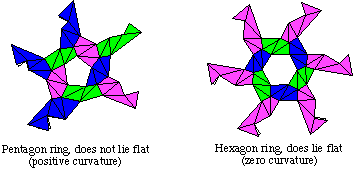So what kind of polyhedra are there that have only pentagon and hexagon faces? Actually, such polyhedra are called Buckyballs or sometimes fullerenes, named after the artist-architect Buckminster Fuller. Such polyhedra were popularized by chemists in the early 1980s because they describe the molecular structure of various carbon molecules.

Fun Project Idea! Write a paper on how Buckyballs can be found in molecules that occur in nature. Does studying Buckyballs using graph theory help the chemists understand them better?

Before we go too much further, let's define Buckyballs formally.

Definition: A Buckyball is a polyhedron with two properties: (1) every face is either a pentagon or a hexagon and (2) each vertex has degree 3.

Exercise: Use Euler's Formula to prove that every Buckyball must have exactly 12 pentagon faces.

That exercise is very surprising! It says that no matter how big a Buckyball we make, we MUST have 12 pentagons, no more, no less. However, we apparently can have as many hexagons as we like.

Definition: A Buckyball is called a spherical Buckyball if its 12 pentagon faces are evenly-spaced on the surface of the Buckyball.

That definition might not seem very rigorous, but it'll do for now. Spherical Buckyballs are also known as geodesic spheres or geodesic domes. The Epcot Center dome, for example, is a spherical Buckyball. So is the soccer ball: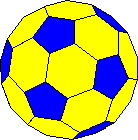Exercise: Draw the planar graph of the soccer ball!

We still don't know how we can even think about inserting more hexagons in between the pentagons to make bigger and bigger spherical Buckyballs. There are many ways to do this, and all of them use the fact that the dual of the dodecahedron is the icosahedron.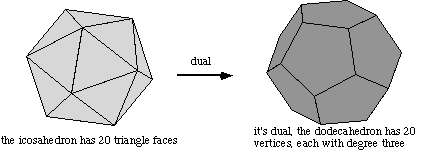That is, we can think of the dodecahedron as the "simplest" Buckyball - all pentagons and no hexagons. To make bigger spherical Buckyballs we'd like to spread the pentagons apart from one another and insert hexagons in between them. HOWEVER, because of the dual nature of the icosahedron and the dodecahedron, we can think of the pentagons as corresponding to the vertices of the icosahedron. If we keep this in mind as we space the pentagons apart, it might help us keep the pentagons "evenly spaced."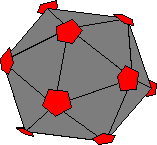So the important thing to realize is this: Each pentagon face of the dodecahedron came from a vertex of the icosahedron. Hmmm ... maybe if we play with the icosahedron a little it can give us larger Buckyballs when we take the dual. This, indeed, is a strategy that works.

Here is what we'll do: take each triangle face of the icosahedron and subdivide it into four smaller triangles, like so...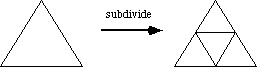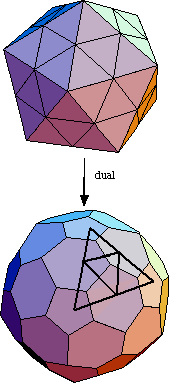To the right is shown what the icosahedron looks like when we subdivide each of its faces in this way. Now, this creates new vertices, but notice that all the vertices have either degree 5 or degree 6. This means that the dual, shown to the lower right, will have only pentagon and hexagon faces.

Furthermore, the only vertices of degree 5 in the subdivided icosahedron are the original corners of the icosahedron, before we subdivided it. This means that the pentagons in the dual will be "spread out" evenly, because the corners of the icosahedron are evenly spread out. Thus we do indeed get a spherical Buckyball when we do all this. (Note: this is not the soccer ball! We'll get to that later.)

Also, this construction gives us an easy way to understand the structure of the Buckyball. Each triangle side of the icosahedron was subdivided, giving us a "piece", or tile of what the whole subdivided icosahedron looks like. The dual of this piece gives us a tile of what this Buckyball looks like, shown below.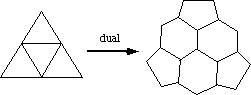These tiles are of great help when trying to construct such Buckyballs using PHiZZ units. You don't have to keep the whole polyhedra in your mind at once. Rather, you can start by making one "piece" and then adding another "piece" to that, and another, and another, keeping in mind that each "piece" corresponds to a triangle face of the icosahedron. The only polyhedral structures that you have to keep in your mind are the icosahedron and one of these tiles!

Try it. This Buckyball has 120 edges, which means that you'll need 120 units to make it. Later, we'll use these tiles as a tool for coloring the units properly with only three colors, but even when made using all one color this object is quite stunning!

Larger and larger Buckyballs can be made using this subdivision method. If we subdivide each triangle of the icosahedron into nine smaller triangles (you do this by dividing each side of the original triangle into thirds, as opposed to halves like we did before), you get the following tiles: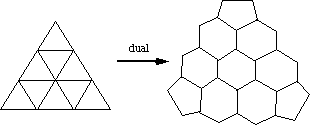Again, you don't have to know exactly what the whole structure looks like to make this larger Buckyball. Each tile looks like the configuration of pentagons and hexagons to the above right, and each piece corresponds to a face of the icosahedron, where the pentagons are fixed at the corners of the icosahedron. This one takes 270 units. Below is a picture of what this Buckyball looks like, made from 270 PhiZZ units.But also notice how in each of these tiles the pentagons are "pointing-in". That is, they're rotated so that one corner points in towards the center of the tile. For this reason, I like to refer to this family of Buckyballs as the pentagons pointing-in, or PPI family of spherical Buckyballs.

The saga of all this continues in Part 2 of these Buckyball notes!